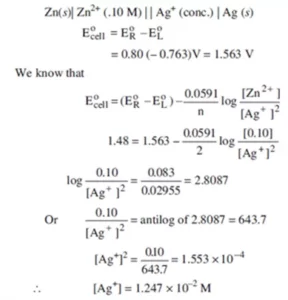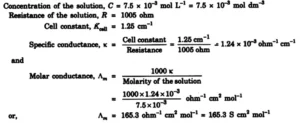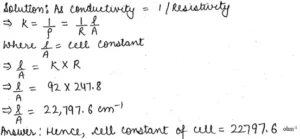# Class 12 Electrochemistry Test Paper – 2 (Solution)# Class 12 Electrochemistry Test Paper – 2 (Solution)

Class 12 Electrochemistry Test Paper – 2 (Solution)

Ans 1. The arrangement of metals and ions in increasing order of their electrode potential values is called electrochemical series. The reduction half-reaction for which the reduction potential is lower than the other will act as an anode and one with greater value will act as a cathode. A reverse reaction will not occur.

Ans 2. Electrochemical cells are used for determining the
a) pH of solutions
b) Solubility product and equilibrium constant
c) In potentiometric titrations

Ans 3. (i) Standard electrode potential and equilibrium constant
E0cell = (2.303RT/nF) log Kc
Where E0cell = Standard electrode potential of cell
R = Gas constant
T = temperature in Kelvin
n = no. of electrons.
Kc = Equilibrium constant

(ii) Standard electrode potential and Gibbs free energy change
∆G0 = -nFE0cell
Where ∆G0 = Change in Gibb’s free energy
n = No. of electrons

Ans 4. The conductivity of an electrolyte depends upon
i) The nature of electrolyte added
ii) Size of the ions produced and their solvation
iii) Concentration of the electrolyte
iv) Temperature

Ans 5. The limiting molar conductivity of an electrolyte(λ0m) is the sum of the limiting molar conductivity of cation (λ0+) and anion (λ0)
Λ0m = ٧+λ0+ + ٧ λ0
Where ٧+ and ٧ are no. of cation and no. of anions formed on dissociation respectively.
Anode: 2H2 (g) + 4OH(aq) → 4H2O (l) + 4e
Cathode: O2 (g) + 2H2O (l) + 4e → 4 OH
Overall cell reaction: 2H2 (g) + O2 (g) → 2H2O (l)

Ans 6.The changes taking place at the electrodes in the electrolysis of Ni (NO3)2 sol using
Ni electrode will be as follows

At Cathode: Ni2+ + 2e → Ni
At Anode: Ni → Ni2+ + 2e
Thus, the amount of Ni deposited on the Cathode from the solution = Ni dissolved from the
Anode. Hence, M of the solution will remain unchanged at the end of the electrolysis.

Ans 7. Metallic Conductance depends upon:
(i) Nature and Structure of the metal
(ii) No of Valance electrons per atom
(iii) Temperature (It decreases with an increase in temperature).
Ionic Conductance depends upon:
(i) The nature of the electrolyte
(ii) Size of ions produced and their solvation
(iii) The nature of the solvent and its viscosity
(iv) The concentration of electrolyte
(v) Temp (It increases with an increase in Temp)

Ans 8.Ans 9. Construction: SHE consists of a platinum electrode coated with platinum black. The electrode is dipped in an acidic solution and pure Hydrogen gas is bubbled through it. The concentration of both the reduced and oxidized forms of Hydrogen is maintained at unity i.e., the pressure of H2 gas is 1bar, and the concentration of Hydrogen ions in the solution is 1molar.
Working: The reaction taking place in SHE is
H+ (aq) + e → ½ H2 (g) At 298 K,
the emf of the cell constructed by taking SHE as anode and another half-cell as cathode gives the reduction potential of the other half cell whereas for a cell constructed by taking SHE as cathode gives the oxidation potential of the other half cells as conventionally the electrode potential of SHE is zero.

Ans 10. E0cell = E0cathode – E0 anode
= E0Ag+ /Ag – E0Cu2+/Cu = 0.80V – (+ 0.34V) = + 0.46V

Class 12 Electrochemistry Test Paper – 2 (Solution)

Ans 11. Na2SO4 → 2 Na+ + SO02-
λ0m (Na2SO4) = 2 [λ0m Na+] + λ0m SO42-
= (2 × 50) + 160 = 260 S cm2 mol-1

Ans 12.  (a) Silver is deposited at the cathode and oxygen is an anode.
Cathode: Ag+ (aq) + 1e → Ag (s)
Anode: 2 H2O (l) → O2 (g) + 4 H+ + 4e
b) H2 gas at the cathode and O2 gas at the anode.
Cathode: H2O (l) + 1e → ½ H2 (g) + OH
Anode: 2 H2O (l) → O2 (g) + 4 H+ (aq) + 4e

Ans 13. Atomic Mass of Ag = 107.92 amu.
Quantity of electricity passed = 0.2 x 3 x 60 x 60 C = 2160 C
Ag+ + e+ → Ag
96500 C deposit Ag = 107.92 g
2160 C will deposit Ag = 107.92 / (96500 × 2160) = 2.4156 g
Volume deposited = Mass / Density = 2.4156 / 10.47 cc = 0.2307 cc
Thickness deposited = Volume / Area = 0.2307 / 800 = 2.88 × 10-4 cm

Ans 14.Ans 15.Ans 16. Anode (oxidation): Cu (S) → Cu2+ (aq) + 2e
Cathode (Reduction): [Ag+ (aq) + e → Ag (s)] × 2
Cell reaction: Cu (s) + 2 Ag+ (aq) → Cu2+ (aq) + 2 Ag (s)
According to Nernst equation
Ecell = E0cell – (0.059/2) log [Products]/[Reactants]
= (0.80-0.40) – (0.059/2) log (0.01)/[Ag+(aq)]2
= 0.46 – (0.059/2) log (0.01)/[Ag+(aq)]2
= [Ag+(aq)] = 1.48 × 10-9 M

Ans 17.  E0cell = E0cathode – E0anode
=> E0cell = E0Ag+ /Ag – E0Fe3+/Fe2+
=> E0cell = 0.80 – 0.76 = 0.04 V
E0cell = (0.059/n) log Kc
Kc = 4.763

Ans 18. Q = I (in amp) × t (in seconds) = 0.5 A × (2 × 60 × 60) s = 3600 C
Ag+ (aq) + 1e → Ag (S)
1 mole of e deposits 1 mol of Ag 1F of charge deposits = 108g of Ag
96500 C deposits = 108g of Ag
3600 C deposits = (108 × 3600)/96500 = 4.026 g of Ag
Volume of silver deposited = mass/density = 4.026 g/10.5g/cm3 = 0.3845 cm3
The thickness of silver deposited = volume/surface area = 0.385 cm3/900 cm2
= 4.272 × 10-4 cm

Ans 19. The metal ions (Zn2+) formed by the loss of electrons will accumulate in one electrode and the negative ions (SO42−) will accumulate in the other. Thus, the solutions will develop charges and the current will stop flowing. Moreover, the inner circuit will not be completed.

Ans 20. (i) As seen from the graph, electrolyte A is a strong electrolyte that is completely ionized in solution. With dilution, the ions are far apart from each other, and hence the molar conductivity increases.
(ii) To determine the value of limiting molar conductivity for electrolyte B, an indirect method based upon Kohlrausch’s law of independent migration of ions is used.

Class 12 Electrochemistry Test Paper – 2 (Solution)